Open In App
Related Articles
• CBSE Class 10 Maths Notes
• CBSE Class 10 Maths Formulas
• NCERT Solutions for Class 10 Maths
• RD Sharma Class 10 Solutions

# Pythagoras Theorem

Pythagoras Theorem, also famously known as the Pythagorean theorem, explains the relationship between the three sides of a right-angled triangle and is the most proven theorem in mathematics. There are well over 371 proofs for the Pythagorean Theorem, one of which is given by American President, James Abram Garfield (who was elected America’s president in 1831), while discussing with a member of Congress he came up with the idea of this proof. Other than James A. Garfield, Eienstien also gave proof for Pythagoras’ theorem.

Pythagoras theorem formula is basically the mathematical expression for the Pythagoras theorem. This formula is used to calculate the third side of the right-angled triangle given the other two sides.

You will get to know everything important related to Pythagoras theorem in this article including Pythagoras theorem formula, proof of Pythagoras theorem as well as its examples.

## What is Pythagoras Theorem?

Pythagoras theorem provides us with the relationship between the sides in a right-angled triangle. A right triangle consists of two legs and a hypotenuse. The two legs meet at a 90° angle, and the hypotenuse is the longest side of the right triangle and is the side opposite the right angle. Observing any right-angled triangle, One of the angles of a right-angled triangle is always 90°, and the square of the hypotenuse is equal to the sum of the squares of the perpendicular and base of the triangle.

## History of Pythagoras Theorem

The history of the Pythagoras Theorem goes back to the ancient Babylon and Egypt eras. It is named after the ancient Greek mathematician and philosopher Pythagoras of Samos, who lived during the 6th century BCE. But the roots of this theorem go to ancient cultures, as it is very likely that Babylonians and Indians used this theorem well before Pythagoras, but its widespread use came into existence after Pythagoras stated it. One of the other reasons this theorem is known as Pythagoras or Pythagorean Theorem is because the disciples of Pythagoras spread knowledge and philosophy of Pythagoras after his death as well.

## Pythagoras Theorem Formula

Pythagoras theorem formula is AC2 = AB2 + BC2, where AB is the perpendicular side, BC is the base, and AC is the hypotenuse side. The Pythagoras equation is applied to any triangle that has one of its angles equal to 90°.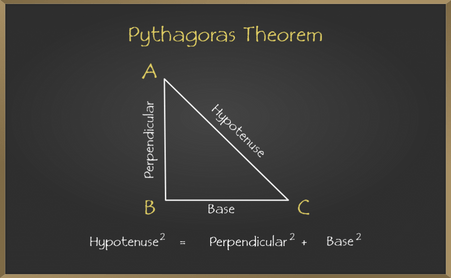Pythagoras formula

The Pythagoras theorem formula or the Pythagorean theorem formula states that in a △ABC, the square of the hypotenuse (AC2) is equal to the sum of the squares of the sides (AB2 + BC2). Here, AB is the perpendicular of the triangle, and BC is the base. The three sides of the right-angled triangle are called the Pythagoras Triplets.

## Derivation of Pythagoras Theorem Formula

Consider a right-angled triangle having sides A, B, and C. Here, AC is the longest side (hypotenuse), and AB and BC are the legs of the triangle. Draw a perpendicular line BD at AC as shown in the figure below,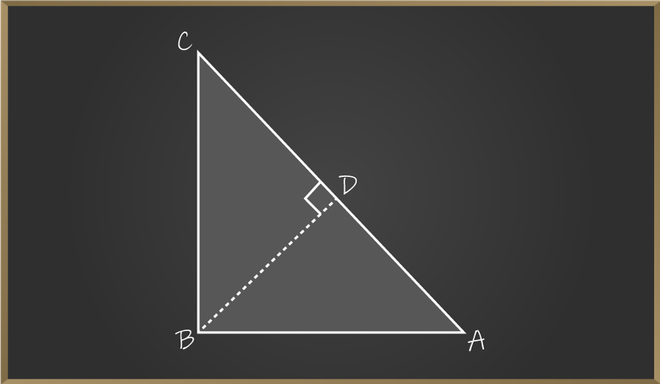Derivation of Pythagoras Theorm

In △ABD and △ACB,

∠A = ∠A (Common angle)

Therefore, we can say △ABD ∼ △ ACB (By AA Similarity)

Similarly, △BDC ∼ △ACB

AB2 = AD × AC ⇢ (1)

And, CD/BC = BC/AC

BC2 = CD × AC ⇢ (2)

AB2 + BC2 = AC × AD + AC × CD

AB2 + BC2 = AC (AD + CD)

AB2 + BC2 = AC × AC

AB2 + BC2 = AC2

Also, AC2 = AB2 + BC2

Hence proved.

## Pythagoras Theorem Proof

Let’s see the traditional way to prove Pythagoras theorem formula which says that the area of the square on the hypotenuse is equal to the sum of the areas of the squares on the two shorter sides. The explanation for Pythagoras theorem can also be seen in a way that the square formed by the hypotenuse side is equal to the sum of the squares formed by the perpendicular side and base side. In the example below, the area formed by side 3 units (c) and 4 units (a) is equal to the area formed by side 5 units (b).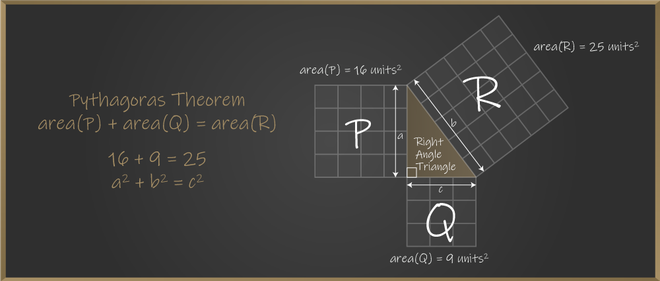## Converse of Pythagoras Theorem

The converse of the Pythagoras theorem is very similar to Pythagoras theorem. To understand this theorem, you should think from the reverse of Pythagoras theorem.

If the square of the length of the longest side of a triangle is equal to the sum of the squares of the other two sides, then the triangle is a right triangle.

### Formula and Proof of Converse Pythagoras Theorem

The formula will be the same as it is just converse of the Pythagoras theorem. According to the statement, we need to prove that the condition occurs, then the triangle should be the right triangle. For that, we need to prove that the opposite angle of the longest side should be 90° if there is a triangle with lengths a, b, and c.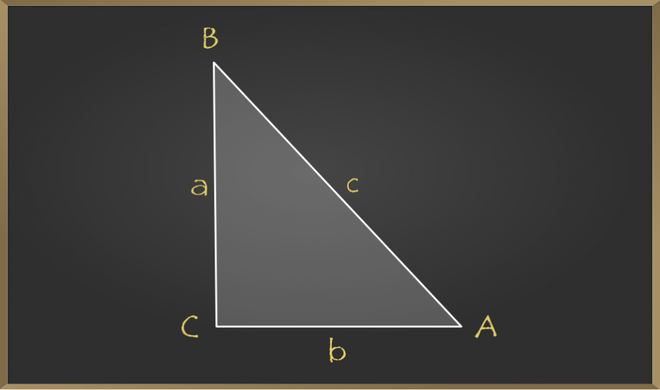We assume that it satisfies c2 = a2 + b2, and by looking into the diagram, we can tell that ∠C = 90°, but to prove it, we require another triangle △EGF, such as AC = EG = b and BC = FG = a.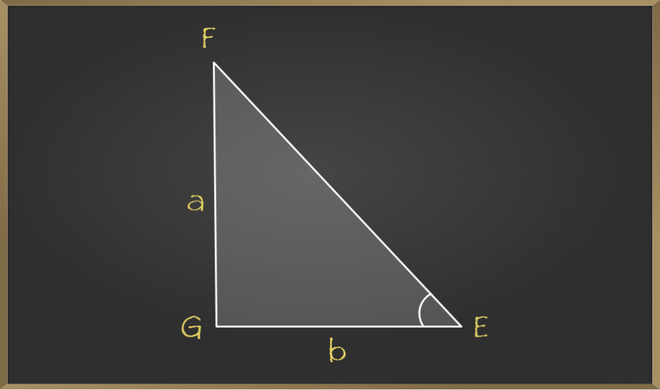In △EGF, by Pythagoras Theorem:

⇒ EF2 = EG2 + FG22 = b2 + a2 ⇢ (1)

In △ABC, by Pythagoras Theorem:

⇒ AB2 = AC2 + BC2 = b2 + a2 ⇢ (2)

From equation (1) and (2), we have;

⇒ EF2 = AB2

⇒ EF = AB

⇒ △ ACB ≅ △EGF (By SSS postulate)

⇒ ∠G is right angle

Thus, △EGF is a right triangle. Hence, we can say that the converse of the Pythagorean theorem also holds.

## Applications of Pythagoras Theorem

The real-life application of Pythagoras theorem can be seen in day-to-day life and in various fields. Below are some of the applications:

• For Navigation, the Pythagoras theorem is calculated in order to find the shortest distance, etc.
• In order to recognize faces in security cameras, Pythagoras theorem is required to understand the distance of the person from the camera.
• In engineering fields, Pythagoras is used to calculate the unknown dimension, say, the diameter of a particular sector.

Related Articles

## Solved Examples on Pythagoras Theorem

Example 1: In the below given right triangle, find the value of y.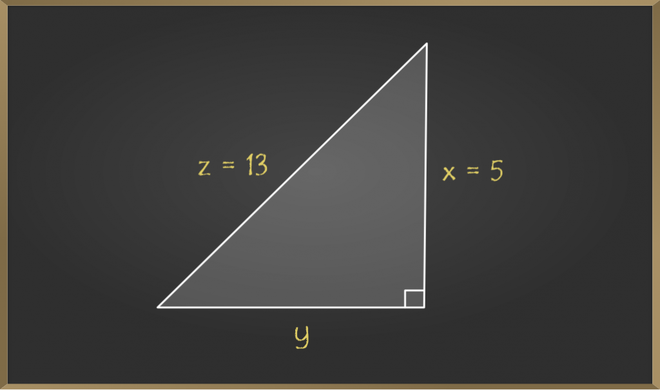Solution:

By the statement of the Pythagoras theorem we get,

⇒ z2 = x2 + y2

Now, substituting the values directly we get,

⇒ 132 = 52 + y2

⇒ 169 = 25 + y2

⇒ y2 = 144

⇒ y = √144 = 12

Example 2: Given a rectangle with a length of 4 cm and breadth of 3 cm. Find the length of the diagonal of the rectangle.

Solution: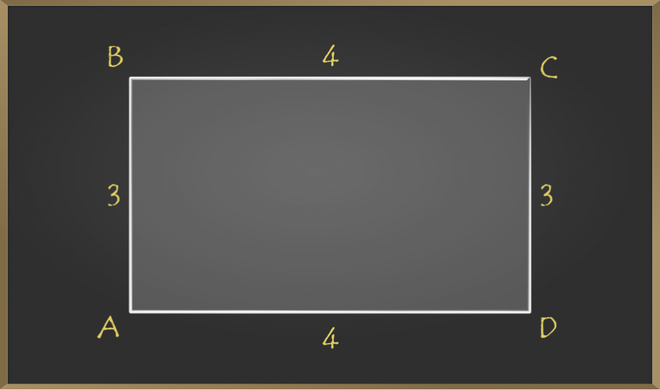In the above diagram length of the rectangle is 4 cm, and the width is 3 cm. Now we have to find the distance between point A to point C or point B to point D. Both give us the same answer because opposite sides are of the same length i.e., AC = BD. Now let’s find the distance between points A and C by drawing an imaginary line.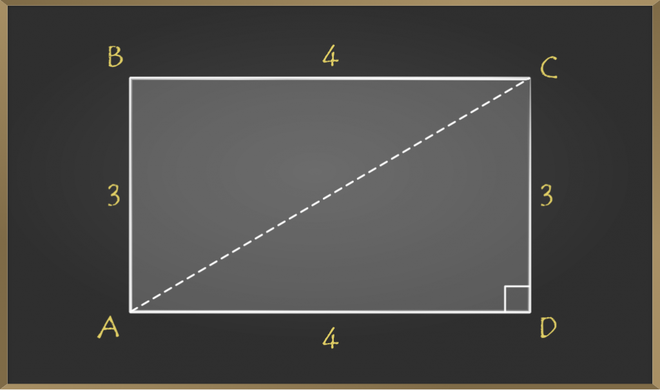Now triangle ACD is a right triangle.

So by the statement of Pythagoras theorem,

⇒ AC2 = AD2 + CD2

⇒ AC2 = 42 + 32

⇒ AC2 = 25

⇒ AC = √25 = 5

Therefore length of the diagonal of given rectangle is 5 cm.

Example 3: The sides of a triangle are 5, 12, and 13. Check whether the given triangle is a right triangle or not.

Solution:

Given,

⇒ a = 5

⇒ b = 12

⇒ c = 13

By using the converse of Pythagorean Theorem,

⇒ a2 + b2 = c2

Substitute the given values in the above equation,

⇒ 132 = 52 + 122

⇒ 169 = 25 + 144

⇒ 169 = 169

So, the given lengths satisfy the above condition.

Therefore, the given triangle is a right triangle.

Example 4: The side of a triangle is of lengths 9 cm, 11 cm, and 6 cm. Is this triangle a right triangle? If so, which side is the hypotenuse?

Solution:

We know that hypotenuse is the longest side. If 9 cm, 11 cm, and 6 cm are the lengths of the angled triangle, then 11 cm will be the hypotenuse.

Using the converse of Pythagoras theorem, we get

⇒ (11)2 = (9)2 + (6)2

⇒ 121 = 81 + 36

⇒ 121 ≠ 117

Since, both the sides are not equal therefore 9 cm, 11 cm and 6 cm are not the side of the right-angled triangle.

## Practice Questions on Pythagoras Theorem

Q1: If the two shorter sides of a right angled triangle measures 14 and 15 cm, find the length of the longest side.

Q2: If the hypotenuse and perpendicular of a right angled triangle are 5 and 4 cm then find the base.

Q3: In a triangular field the sides measures 24 cm, 7 cm and 25 cm then prove that field is the form of a right triangle.

Q4: A wall is of 12 m height and a ladder of 13 m is placed against it touching its top. Find the distance between the foot of the ladder and the wall.

## FAQs on Pythagoras Theorem

### 1. Who discovered Pythagoras Theorem?

The Greek mathematician and philosopher Pythagoras of Samos (commonly known as Pythagoas) is credited with the discovery of Pythagoras’ Theorem. But scientists also found inscriptions of the same theorem in the ruins of ancient Egypt and Babylon, but their use of the theorem was not widely publicized until Pythagoras stated it.

### 2. What is Pythagoras Theorem in math?

The Pythagoras theorem provides us with the relationship between the sides in a right-angled triangle. The square of the hypotenuse is equal to the sum of the sides of the perpendicular and base. It can be written as:

c2 = a2 + b2

Where c is the hypotenuse, a and b are the legs of the right-angled triangle.

### 3. What is the Converse of Pythagoras Theorem?

The converse of Pythagoras theorem states that if the square of the length of the longest side of a triangle is equal to the sum of the squares of the other two sides, then the triangle is a right triangle.

### 4. What are the applications of Pythagoras Theorem?

The applications of the Pythagoras theorem are in various fields: